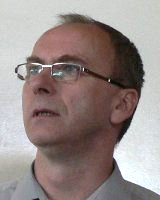# prof. RNDr. Josef Tkadlec, CSc.Professor
22435 5109
JP:B-509

Teaching experience: Lectures in Linear Algebra, Calculus, Numerical Analysis, Differential Equations. Seminars in Linear Algebra, Mathematical Logics, Calculus, Real and Complex Analysis, Probability Theory, Numerical Analysis, Differential Equations.

Research interests: Algebraic and set-theoretical structures related to quantum theories (quantum logics, orthoposets, effect algebras), state spaces, set-representations, fuzzy logics.